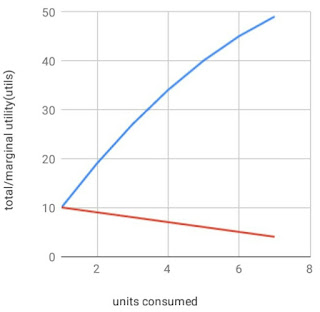# UTILITY, TOTAL UTILITY, MARGINAL UTILITY AND AVERAGE UTILITY

0

The theory of demand starts with the examination of the behaviour of consumers. Hence, it is worthwhile that we discuss Utility.

Utility refers to the pleasure or satisfaction that an individual derived from economic activity.

It can also be defined as the pleasure or satisfaction that an individual gets from consuming units of goods.

The utility is subjective as it varies from person to person, time to time and place to place.

The concept of utility assumes that utility can be measured using an artificial construct called utils.

Various utility analysis is used by economics. These include total utility, Marginal utility and Average utility.

### Total utility

This is the total satisfaction derived from consuming all available units of goods in a given period.

The utility is additive. As a result, total utility increases (albeit in a decreasing manner) as consumers consume more units of goods.

If by consuming the first orange, Samuel derives 9 utils of satisfaction, 8 utils from consuming the second orange, and 7 utils from the third orange.

It follows that Samuel's total utility is $9+8+7=24$.

Another way of thinking about total utility is that it is the sum of all marginal utilities associated with consuming each unit of goods.

That is, total utility is:
$$TU=MU_1+MU_2+MU_3...MU_n$$

### Average utility

Average utility is the per-unit utility that a consumer derived from consuming units of a good.

It is obtained by dividing total utility by units consumed. If we are to represent this mathematically, it will be like this:

$$AU=\frac{TU}{Q}$$

Where $TU$=Total utility
and $Q$= total units consumed.

For example, if a consumer derived a total utility of 40 from consuming 8 oranges. Then, the Average utility is:

$AU=\frac{40}{8}$

$AU=5$

### Marginal utility (MU)

Marginal utility is the additional utility derived from consuming an additional unit of goods.

It is the ratio of change in total utility to the change in units of goods sold.

Mathematically, MU is represented as:

$$MU=\frac{∆TU}{∆Q}$$

Where $∆TU$ is a change in total utility and

$∆Q$ is a change in total units consumed

Perhaps the best way to explain utility is to represents it on a table.

 Units Tu (utils) Mu (utils) Au(utils) 1 10 10 10 2 19 9 9.5 3 27 8 9 4 34 7 8.5 5 40 6 8 6 45 5 7.5 7 49 4 7

If we were to represent the above information on a graph, it will appear like this.## Marginal Utility and Total Utility curve

As the consumer consumes more units of goods, the total utility increases while the marginal utility keeps decreasing. In other words, the total utility increases as marginal utility decreases.

Economists call this pattern the law of diminishing marginal utility. This law state that: at any given period, the marginal utility will decrease as consumption is increased.

In other words, the marginal utility of the first unit will be greater than the marginal utility of the second and so on. There may come a point when the marginal utility will be zero, indicating dissatisfaction or disutility.

To better understand the law of diminishing marginal utility. Let's analyze a real-life example of ice cream. Consuming your first ice cream will give you the maximum satisfaction possible.

If you consume a second ice cream you would also get some satisfaction. However, the satisfaction you obtained from the second will likely be lesser than the first.

Consuming the third ice cream would give you even lesser satisfaction. After the sixth ice cream, you would probably feel disgusted by the idea of Ice-cream.

However, we must realize that the law of diminishing marginal utility has few limitations, which are highlighted below:

1. Good should be homogenous: The law of diminishing marginal utility does not hold if the goods are heterogeneous.

For this law to hold, we assume that goods are homogenous in that they are similar in size and shape.

2. No time gap: For this law to hold good, there must be no time gap in consumption.

The law of diminishing marginal utility will be falsified if there is a gap in consumption.

For example, if we take the second orange after a long time of consuming the first orange, the marginal utility will rise instead of falling.

3. Does not hold for money: The law of diminishing marginal utility does not hold for money.

It is said that the more money you have, the more of it you will want! Or is it wrong? 😎

Related posts

## Relationship Between Total Utility and Marginal Utility

The relationship between marginal utility and total utility is such that:
1. Total utility rises when marginal utility is positive. This is the initial case when units of goods are consumed.

2. Total utility is constant when marginal utility is zero. This is the case when an individual is satisfied to the maximum. Any further consumption will lead to dissatisfaction.

3. Total utility decreases when marginal utility is negative. This is the case with dissatisfaction and disutility

As can be seen from the diagram above, the point where total utility is maximum and marginal utility is zero is called the saturation point.

There you have it! In our next post, we will learn about budget constraints.

Meanwhile, if you have got questions regarding this, do well to ask our telegram community

Tags